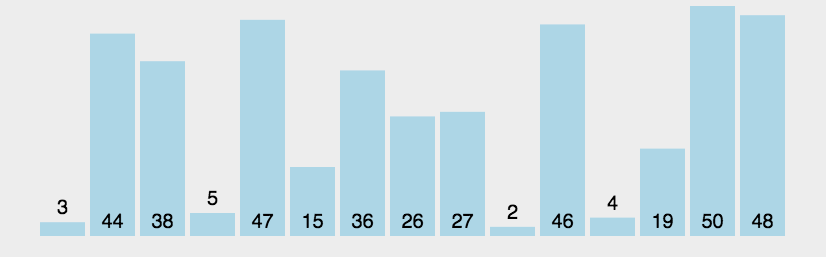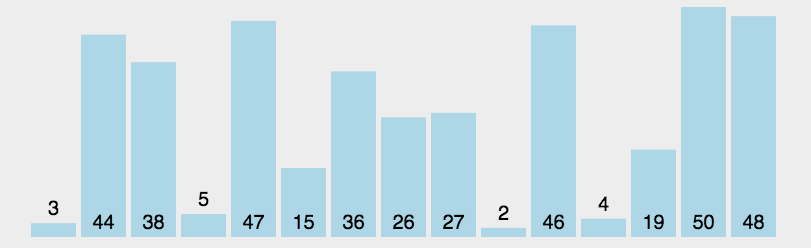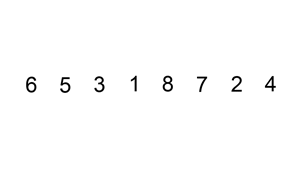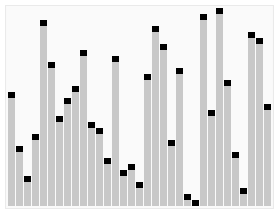menu 首页 标签 归档 视频 关于# 冒泡排序

1. 比较相邻的两个数据，如果前者大于后者，则进行交换位置，否则不交换
2. 把每一个元素与相邻的数据做比较，完成后，最后的一个元素是最大数
3. 重复上面过程，直到排序怕完成``````int *BubbleSort(int a[],int size)
&#123;
//默认已经完成排序
bool isSort = true;
for (int i = 0; i < size-1; i++)
&#123;
//每次默认已经排序完成
isSort = true;
for (int j = 0; j < size-1-i; j++)
&#123;
if (a[j] > a[j + 1])
&#123;
a[j] = a[j] ^ a[j + 1];
a[j + 1] = a[j] ^ a[j + 1];
a[j] = a[j] ^ a[j + 1];
//当发生交换时表明排序未完成
isSort = false;
&#125;
&#125;
//判断如果排序完成：跳出循环
if (isSort)
break;
&#125;
return a;
&#125;
``````

# 选择排序

1. 每一趟从待排序的记录中选出最小的元素，顺序放在已排好序的序列最后，直到全部记录排序完毕。``````int *SelectionSort(int a[], int size)
&#123;
for (int i = 0; i < size; i++)
&#123;
//设置当前索引对应值为最小值
int min_index = i;
for (int j = i; j < size; j++)
&#123;
//如果有比记录的最小值小，则记录下来
if (a[j] < a[min_index])
min_index = j;
&#125;
if (min_index != i)
&#123;
//数值交换
Swap(a[min_index], a[i]);
&#125;

&#125;
return a;
&#125;
``````

# 插入排序

1. 从第一个元素开始，该元素可以认为已经被排序；
2. 取出下一个元素，在已经排序的元素序列中从后向前扫描；
3. 如果该元素（已排序）大于新元素，将该元素移到下一位置；
4. 重复步骤3，直到找到已排序的元素小于或者等于新元素的位置；
5. 将新元素插入到该位置后；
6. 重复步骤2~5。``````int *InsertionSort(int a[], int size)
&#123;
for (int i = 1; i < size; i++)
&#123;
int temp = a[i];
int index = i-1;
while (index>=0 && temp<a[index])
&#123;
a[index + 1] = a[index];
--index;
&#125;
a[index + 1] = temp;
&#125;
return a;
&#125;
``````

# 折半插入排序

``````void HalfInsertionSort(int a[], int size)
&#123;
for (int i = 1; i < size; i++)
&#123;
int l = 0;
int h = size - 1;
int temp = a[i];
while (l <= h)
&#123;
int mid = (l + h) / 2;
if (temp <= a[mid])
h = mid - 1;
else
l = mid + 1;
&#125;

for (int j = i-1; j >= l; j--)
a[j+1] = a[j];
a[l] = temp;
&#125;
&#125;
``````

# 快速排序

1. 先从数列中取出一个数作为基准数。
2. 分区过程，将比这个数大的数全放到它的右边，小于或等于它的数全放到它的左边。
3. 再对左右区间重复第二步，直到各区间只有一个数。``````void QuickSort(int a[], int low, int high)
&#123;
if (low < high)
&#123;
int l = low;
int h = high;
//定义支点为第一个
int pivot = a[low];

//当i==j的时候，说明我们找到了一个中间位置，这个中间位置就是基准数应该所在的位置
while (l<h)
&#123;
//从后往前比较，找一个比pivot小（或者=）的数字，放在a[l]所在位置
while (l<h && pivot<a[h])
&#123;
h--;
&#125;
a[l] = a[h];
//从前往后比较，找一个比pivot大的数字，放在a[h]所在位置
while (l<h && a[l]<pivot)
&#123;
l++;
&#125;
a[h] = a[l];
&#125;
//放入基准数
a[l] = pivot;
//此时在pivot所在位置，分为左右两部分
//通过递归方式，直到完成排序
QuickSort(a, low, l - 1);
QuickSort(a, l + 1, high);
&#125;
&#125;
``````

# 参考

siki 快速排序

GIF 动态图来源于网络

---2018.10.14arrow_upward